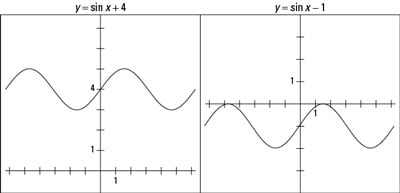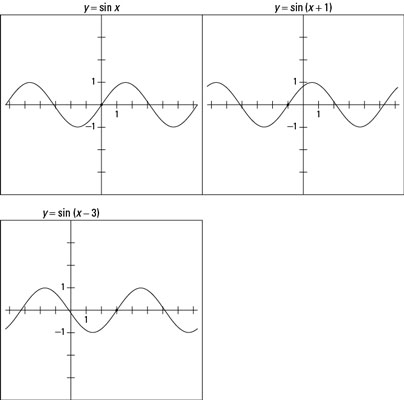##### Trigonometry For DummiesPlaying around with the amplitude and period of the sine curve can result in some interesting changes to the basic curve on a graph. That curve is still recognizable, though. You can see the rolling, smooth curve crossing back and forth over a middle line.

In addition to those changes, you have two other options for altering the sine curve — shifting the curve up or down, or sideways. These shifts are called translations of the curve.

## Sliding a function up or down on a graph

You can move a sine curve up or down by simply adding or subtracting a number from the equation of the curve. For example, the graph of y = sin x + 4 moves the whole curve up 4 units, with the sine curve crossing back and forth over the line y = 4. On the other hand, the graph of y = sin x – 1 slides everything down 1 unit. The following figure shows what the two graphs look like.The graphs of y = sin x + 4 and y = sin x – 1.

As you can see, the basic shape of the sine curve is still recognizable — the curves are just shifted up or down on the coordinate plane.

## Sliding a function left or right on a graph

By adding or subtracting a number from the angle (variable) in a sine equation, you can move the curve to the left or right of its usual position. A shift, or translation, of 90 degrees can change the sine curve to the cosine curve. But the translation of the sine itself is important: Shifting the curve left or right can change the places that the curve crosses the x-axis or some other horizontal line.

For example, the graph of y = sin (x + 1) results in the usual sine curve slid 1 unit to the left, and the graph of y = sin (x – 3) slides it 3 units to the right. The below figure shows the graphs of the original sine equation and these two shifted equations.Comparing the graphs of y = sin x, y = sin (x + 1), and y = sin (x – 3).

Take a look at the point marked on each graph in the above figure. This point illustrates how an intercept (where the curve crosses an axis) shifts on the graph when you add or subtract a number from the angle variable.

Note the difference between adding or subtracting a number to the function and adding or subtracting a number to the angle measure. These operations affect the curve differently, as you can see by comparing the preceding figures.

y = sin x + 2: Adding 2 to the function raises the curve by 2 units.

y = sin (x + 2): Adding 2 to the angle variable shifts the curve 2 units to the left.